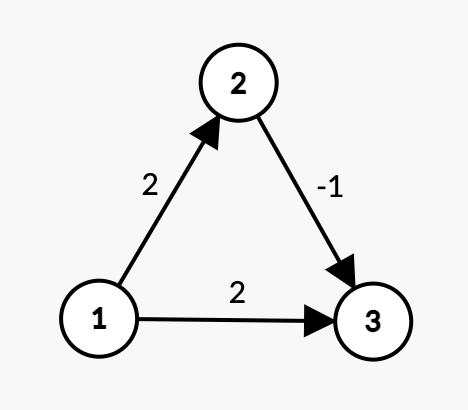New update is available. Click here to update.

# Floyd Warshall

Last Updated: 23 Jul, 2021
Difficulty: Moderate

## PROBLEM STATEMENT

#### Example :``````3 3 1 3
1 2 2
1 3 2
2 3 -1
In the above graph, the length of the shortest path between vertex 1 and vertex 3 is 1->2->3 with a cost of 2 - 1 = 1.
``````

#### Note :

``````It's guaranteed that the graph doesn't contain self-loops and multiple edges. Also the graph does not contain negative weight cycles.
``````

#### Input Format :

``````The first line of input contains an integer ‘T’ denoting the number of test cases. Then each test case follows.

The first line of each test case contains four single space-separated integers ‘N’,  ‘M’ , ‘src’ and ‘dest’ denoting the number of vertices, the number of edges in the directed graph the source vertex and the destination vertex respectively.

The next ‘M’ lines each contain three single space-separated integers ‘u’, ‘v’, and ‘w’, denoting an edge from vertex ‘u’ to vertex ‘v’, having weight ‘w’.
``````

#### Output Format:

``````For each test case, return an integer denoting the length of the shortest path from ‘src’ to ‘dest’. If no path is possible return 10^9.
``````
##### Note:
``````You do not need to print anything, it has already been taken care of. Just implement the given function.
``````

#### Constraints :

``````1 <= T <= 10
1 <= N <= 50
1 <= M <= 300
1 <= src, dest <= N
1 <= u,v <= N
-10^5 <= w <= 10^5

Time Limit: 1 sec
``````